# Myers差分算法分析

• 论文地址
• 摘要
• 定义
• 编辑图
• 解决方案
• 概念
• 贪婪算法
• 最优坐标
• snake坐标组
• 算法实现（伪代码）

## 论文地址

paper论文地址： https://neil.fraser.name/writing/diff/myers.pdf

## 定义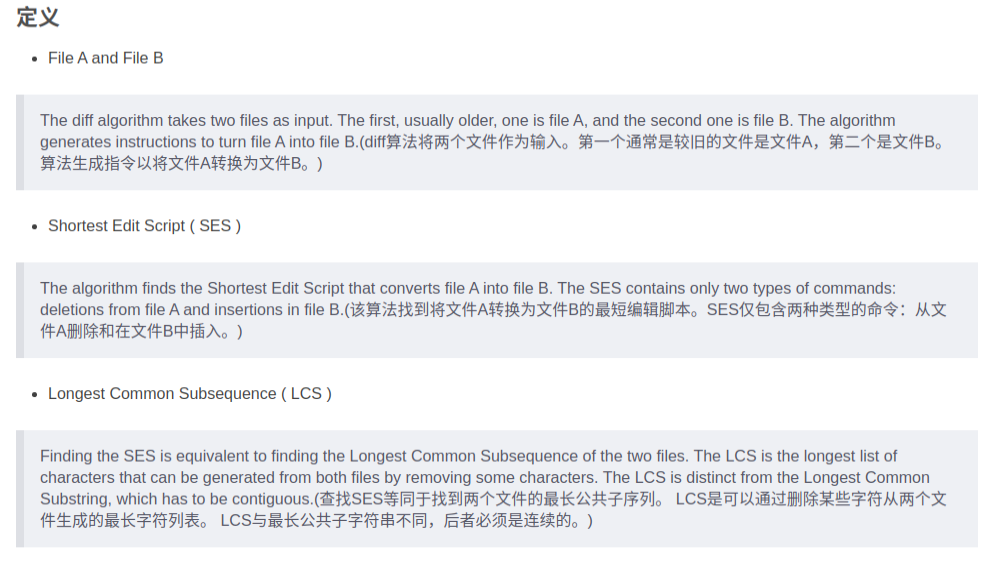## 编辑图

A []的长度为N，B []的长度为M。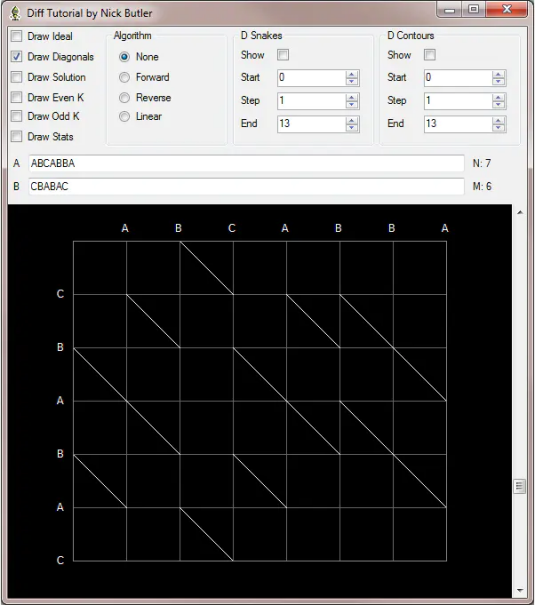## 解决方案

（1）依赖于子问题的最优解
（2）子问题重叠
（3）问题存在边界
（4）子问题独立

Myers差分解决方案：

LCS是轨迹中的对角线，SES是轨迹中的水平和垂直移动。例如，LCS的长度为4个字符，SES的长度为5个差异。## 概念

snake : 一条snake代表走一步。例如从(0,0)->(0,1) / (0,0)->(1,0) / (0,1)->(0,2)->(2,4) 这分别为三条snake，走对角线不计入步数。

k line: 定义 k = x - y （好吧，我习惯写成 y = x - k，这是相同斜率的平行斜线组成的一个集合)

d contour: 走一步算一个 d

## 贪婪算法

1. 路径的终点必然在k线上。迭代进行，所以k线的上一步操作是k+1向下移动或者k-1向右移动；

2. 计算连续的d每条k线上最远的到达路径（偶数d的端点在偶数k线，奇数类似）；

3. 路径到达右下角结束；

k line：棕色线是k的奇数值的k条线。黄线是k的偶数值的k线。

snake：深蓝色的线条是蛇。红蛇显示溶液痕迹。

d contours：淡蓝色的线是差异轮廓。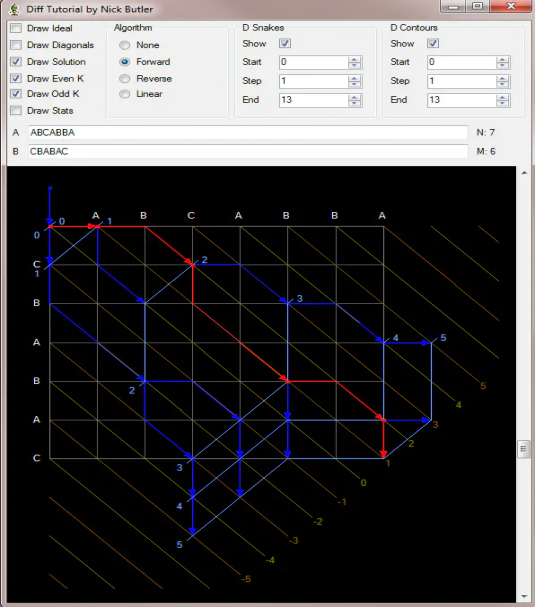for ( int d = 0 ; d <= N + M ; d++ )

for ( int k = -d ; k <= d ; k += 2 )

## 最优坐标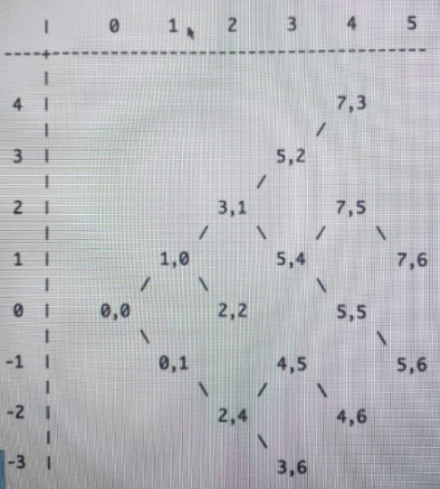## snake坐标组

k = -3：这种情况下，只有当k = -2，d = 2时，向下移动一步(k = -4, d = 2这种情况不存在)。所以，我们可以这么来走，从(2,4)点开始向下走到(2,5),由于(2,5)和(3,6)之间存在一个对角线，可以走到(3,6)。所以着整个snake是：(2,4) -> (2,5) -> (3,6)。

k = -1：有两种情况需要来讨论：分别是k = 0，d = 2向下走；k = -2 ，d = 2向右走。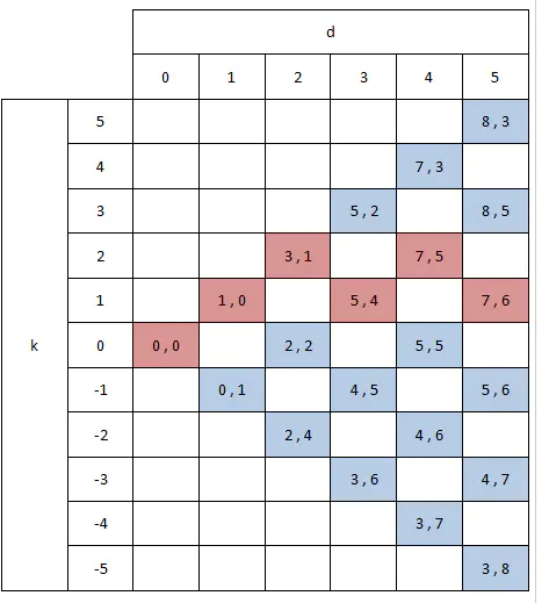## 算法实现（伪代码）

``````//新建集合，用k值保存对应的x
v[ 1 ] = 0;
//步数递增
for (int d= 0; d <= N+M ;d++){
//每一步下面的各个k 的取值循环，
for( int k = -d ; k <= d ; k+=2){
// 是否向下
bool down = ( k== - d || (k !=d && v[k-1] < v[ k+1] ));
//得到上次移动的k,如果这次向下，上次的kPrev = k+1;
int kPrev = down ? k+1 : k -1;
//起点，因为每次移动保存是 k+2 ,所以如果这次的index全是奇数，上一次就全是偶数，在一个数组里面不会进行覆盖
int xStart = v[kPrev]
int yStart = xStart - v[kPrev]
//中点，之前说的，如果没有遇到斜线，它是等于终点的
int xMid = down ? xStrat : xStart +1;
int yMid = xMid - k；
//终点，没有遇到斜线的样子
int xEnd = xMid;
int yEnd = yMid;
//斜线数量
int s= 0
//这就是遇到斜线，只有数据相等，才会是斜线，然后，x,y 各加一
while(xEnd < N && yEnd <M && A[xEnd] ==B[yEnd]){
xEnd++;
yEnd++;
s++;
}
// 保存到数组
v[k] = xEnd;
//到了最终点（N,M）,找到了最短步数的方案
if(xEnD >= N && yEnd > = M){
//跳出循环
}
}
}
``````